﻿ 超大型集装箱船载荷响应特性的数值研究
 舰船科学技术2019, Vol. 41Issue (3): 62-67PDF

The numerical research for wave load response characteristic of ultra large container
PENG Ya-kang, WANG Wei-fei, HAN Yu, QIU Wei-qiang, ZHANG Zhi-kang
Marine Design and Research Institute of China, Shanghai 200011, China
Abstract: Compared with bulk carriers, oil tankers and so on, the springing of ultra large container ships, especially the coupling between bending and torsion, is very obvious. This paper mainly studies the effect of elastic effect on the numerical calculation of wave loads on the target ship. In this paper, the finite element method is used to calculate the bending-torsion coupling modes and the transfer matrix method is used to calculate bending - torsional uncoupling modes respectively. The paper analyses the wave load response of an ultra large container which can carry twenty thousand containers basing on 3D-hyroelastic theory and under the condition of including the bend-torsion coupling phenomenon and the calculation results are compared with those based on rigid body assumption. The paper comes to the conclusion that the phenomenon of bend-torsion coupling and springing cannot be ignored when calculating the wave loads of an ultra large container ship by means of comparing the results between the wave loads under the coupling of bending and torsion and the wave loads under the uncoupling of bending and torsion.
Key words: ultra large container ships     springing     bending-torsion coupling modes
0 引　言

Malenica等在利用频域格林函数法计算水动力的基础上，结合有限元模态分析结果计算了集装箱船所受波浪载荷，并且最终发布了集装箱船的波浪载荷计算商业软件Homer，取得很大成功。

1 理论基础

 \begin{aligned} \left[ { a} \right]\!{\ddot p_r}({ t}) \!\!+\!\! \left[ { b} \right]\!{\dot p_{ r}}({ t}) \!\!+\!\! \left[ { c} \right]{{ p}{_{ r}}}(t) \!\!=\!\!\! {F_{Fluid}}(t) \text{，} \!\!\!\!{r \!\!=\!\!\! 1,2 \cdots ,n} \text{。} \end{aligned} (1)

${F_{Fluid}}(t)$ 即为广义流体力，可以分解为

 ${F_{Fluid}}(t) = {F_I}(t) + {F_D}(t) + {F_R}(t) + {F_S}(t) \text{，}$ (2)

 $[ - {\omega ^2}(\left[ a \right] + \left[ A \right]) + i\omega \left( {\left[ b \right] + \left[ B \right]} \right) + (\left[ c \right] + \left[ C \right])]\left\{ {{p_a}} \right\} = \left\{ F \right\} \text{，}$ (3)

 $\left\{ {\begin{array}{*{20}{c}} {w(x,t) = {e^{ - i{\omega _e}t}}\sum\limits_{r = 0}^m {{p_{ra}}{w_r}(x),} } \\ {M(x,t) = {e^{ - i{\omega _e}t}}\sum\limits_{r = 0}^m {{p_{ra}}{M_r}(x),} } \\ {V(x,t) = {e^{ - i{\omega _e}t}}\sum\limits_{r = 0}^m {{p_{ra}}{V_r}(x)} }{\text{。}} \end{array}} \right.$ (4)

2 模态分析表 1 目标船主要参数 Tab.1 Main parameters of target ship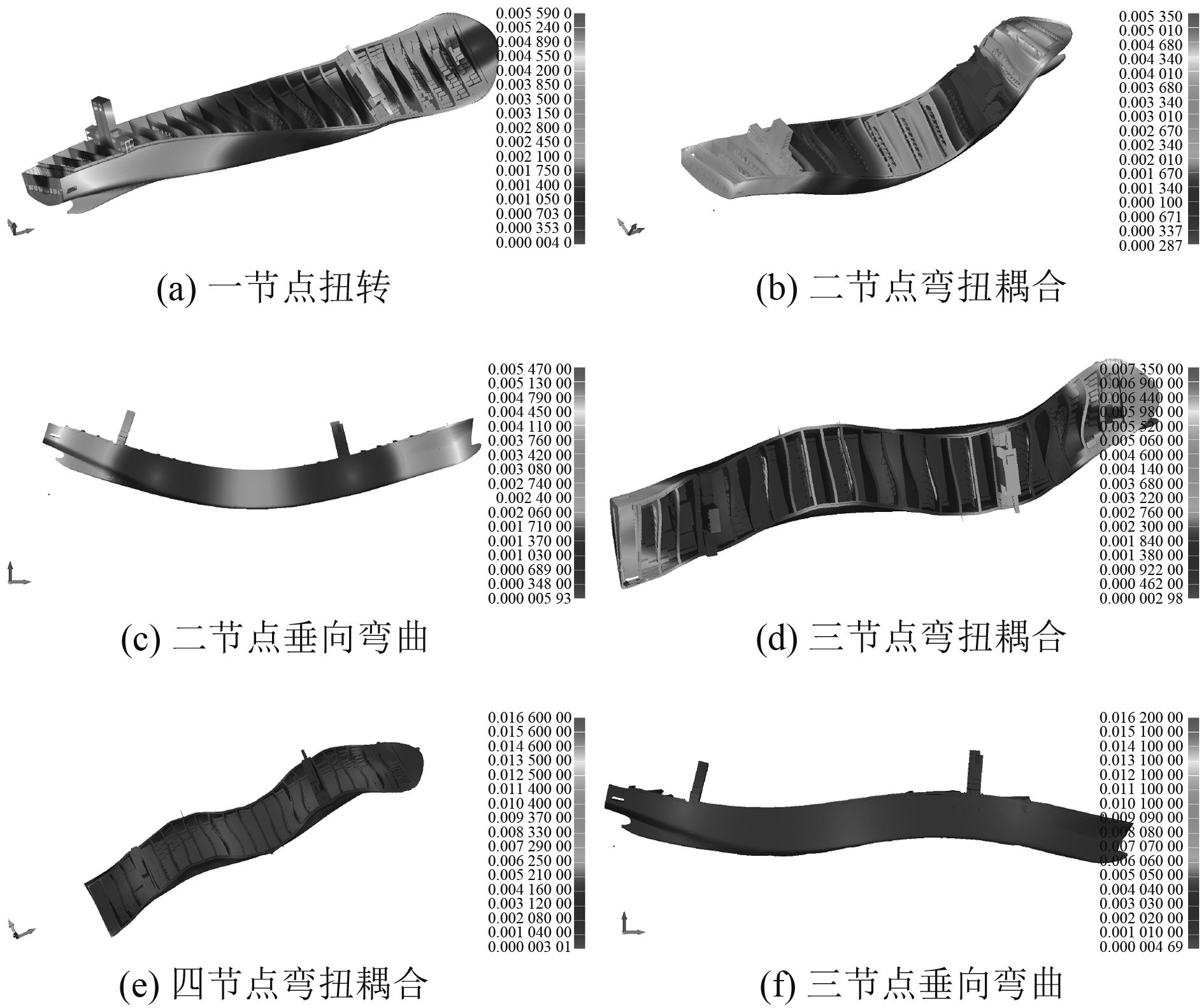图 1 振动模态的有限元分析结果 Fig. 1 Vibration mode results from finite element analysis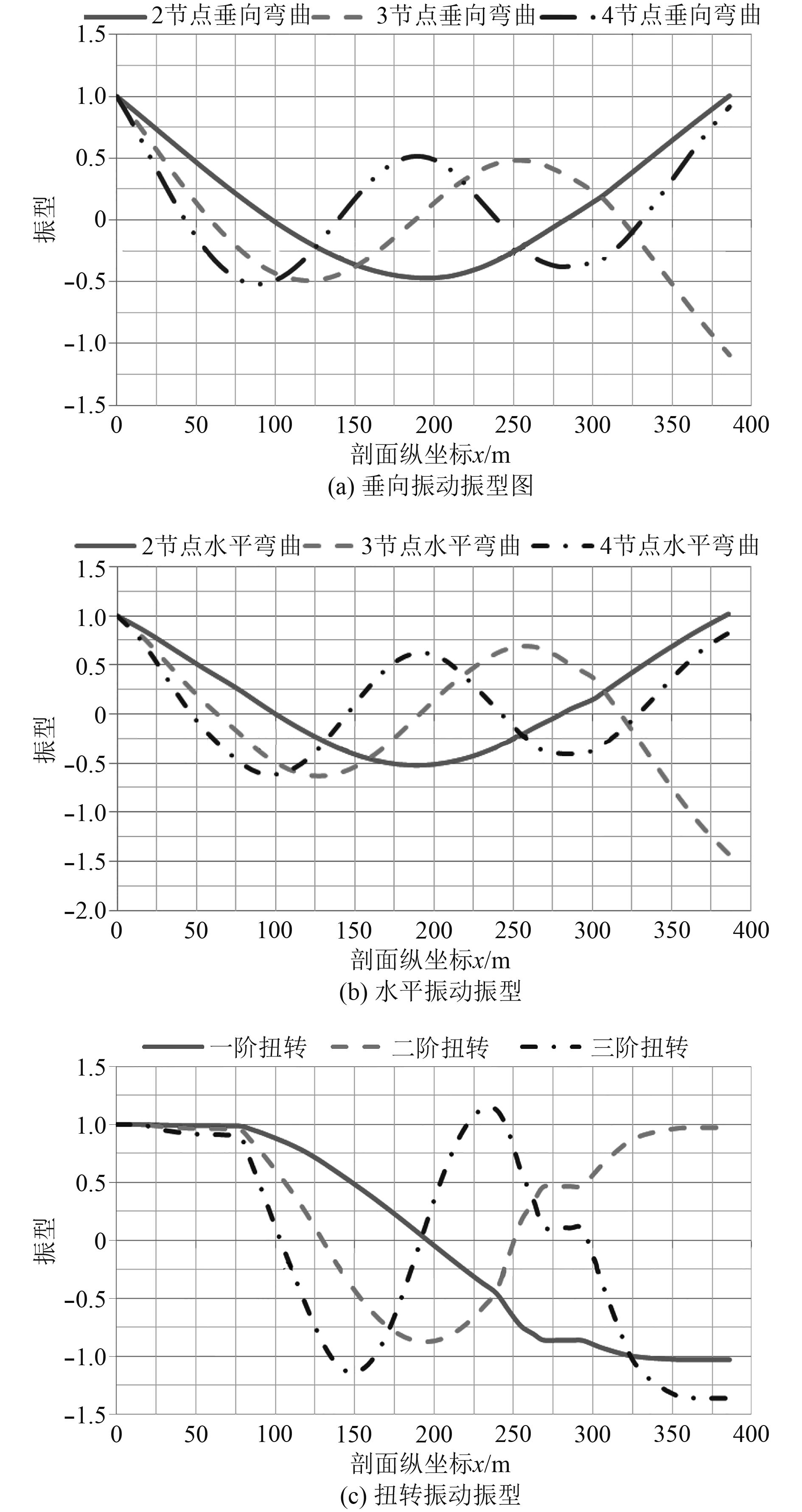图 2 振动模态的迁移矩阵法分析结果 Fig. 2 Vibration mode results from Transfer matrix method表 2 模态计算结果对比分析 Tab.2 Mode results from FE analysis

3 载荷响应特性研究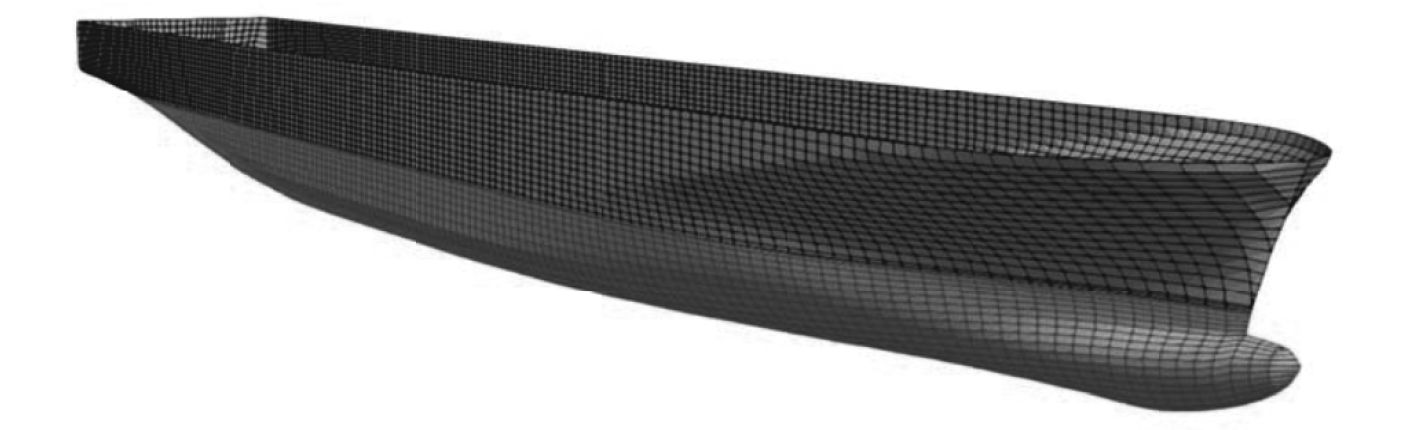图 3 水动力网格 Fig. 3 Hydrodynamic mesh表 3 波浪载荷计算参数 Tab.3 Input parameters of wave loads calculation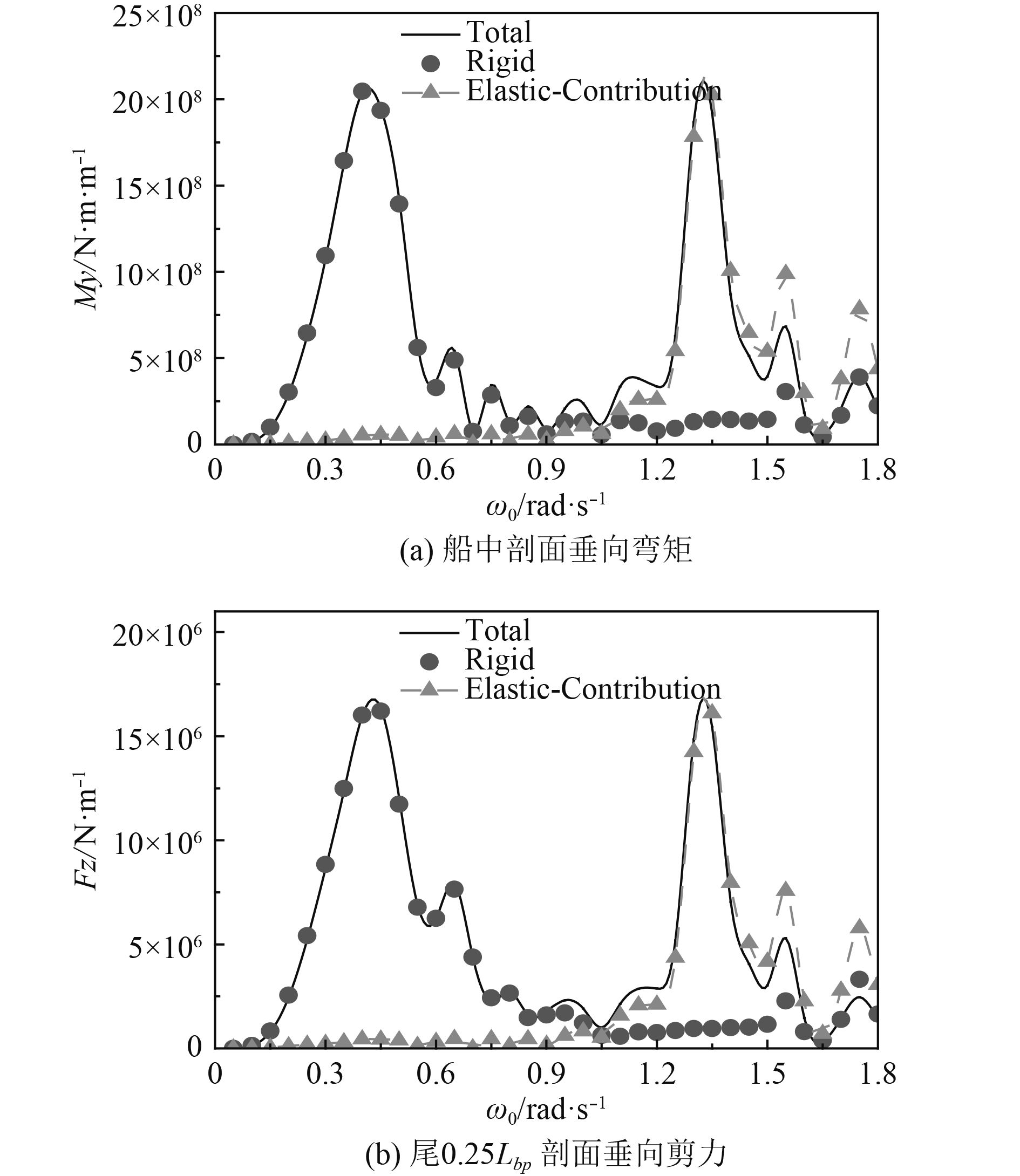图 4 垂向载荷数值计算结果 Fig. 4 Numerical results of vertical wave loads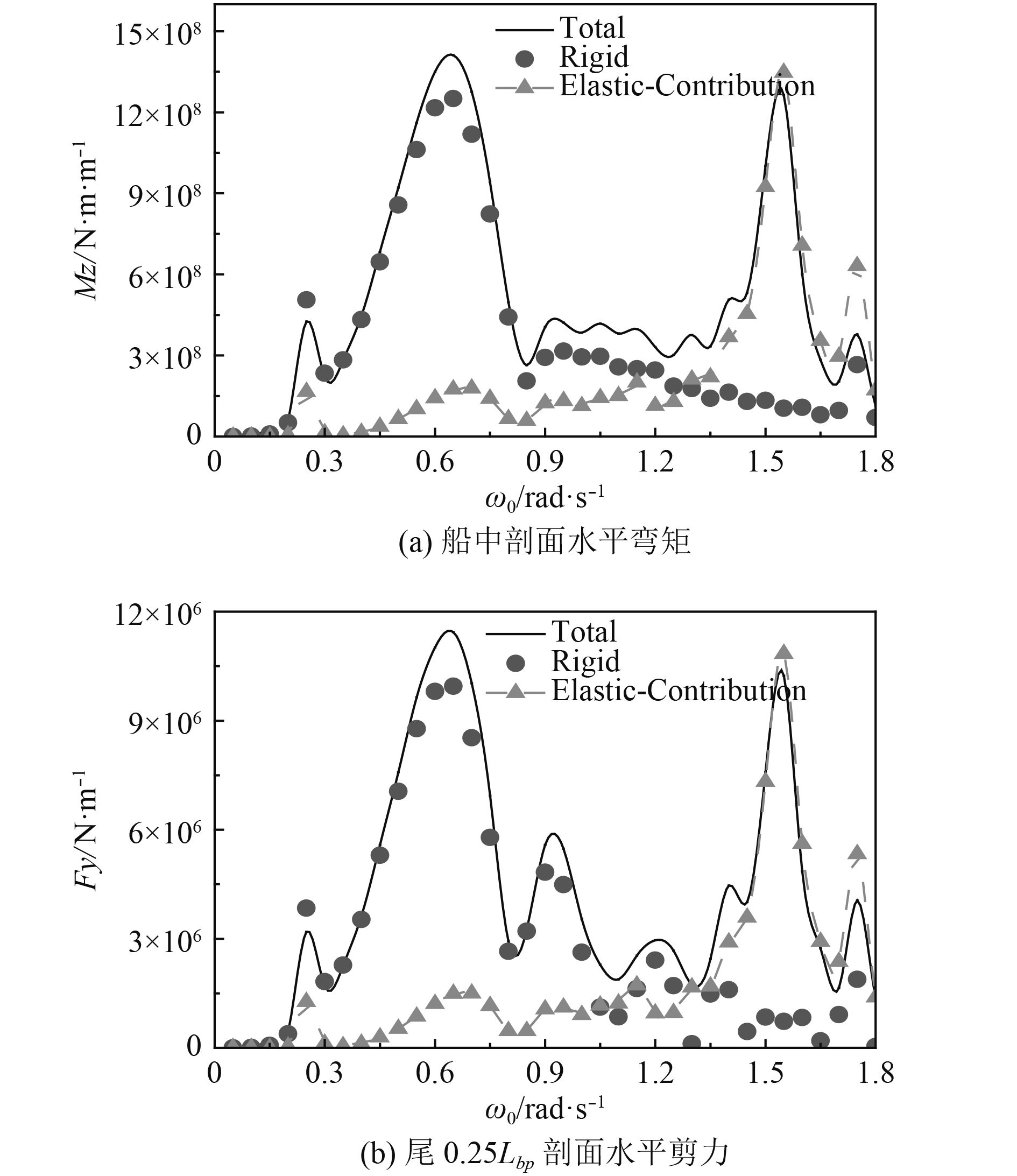图 5 水平载荷数值计算结果 Fig. 5 Numerical results of horizontal wave loads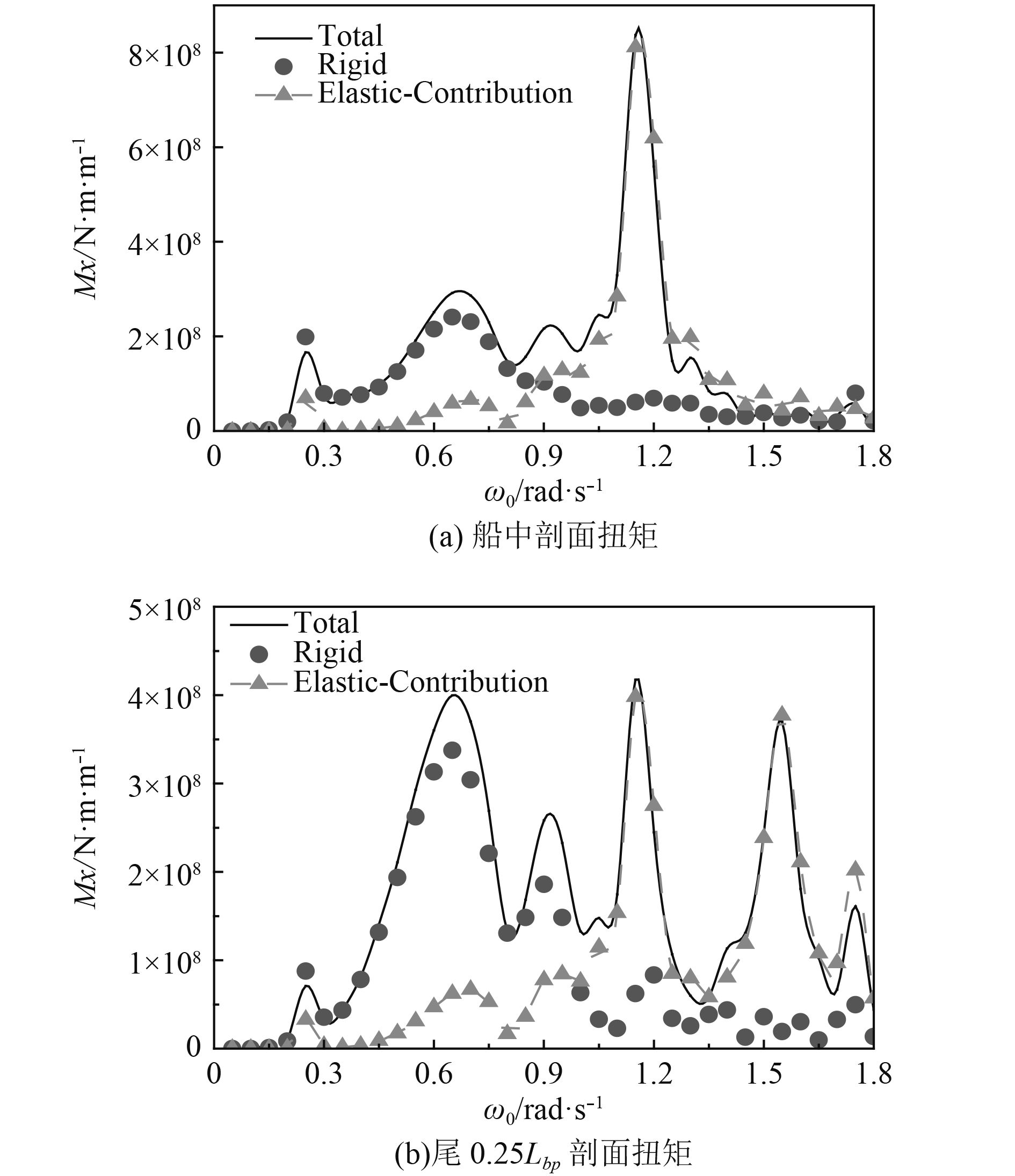图 6 扭矩的数值计算结果 Fig. 6 Numerical results of torque

4 弯扭耦合效应对超大型集装箱船的影响研究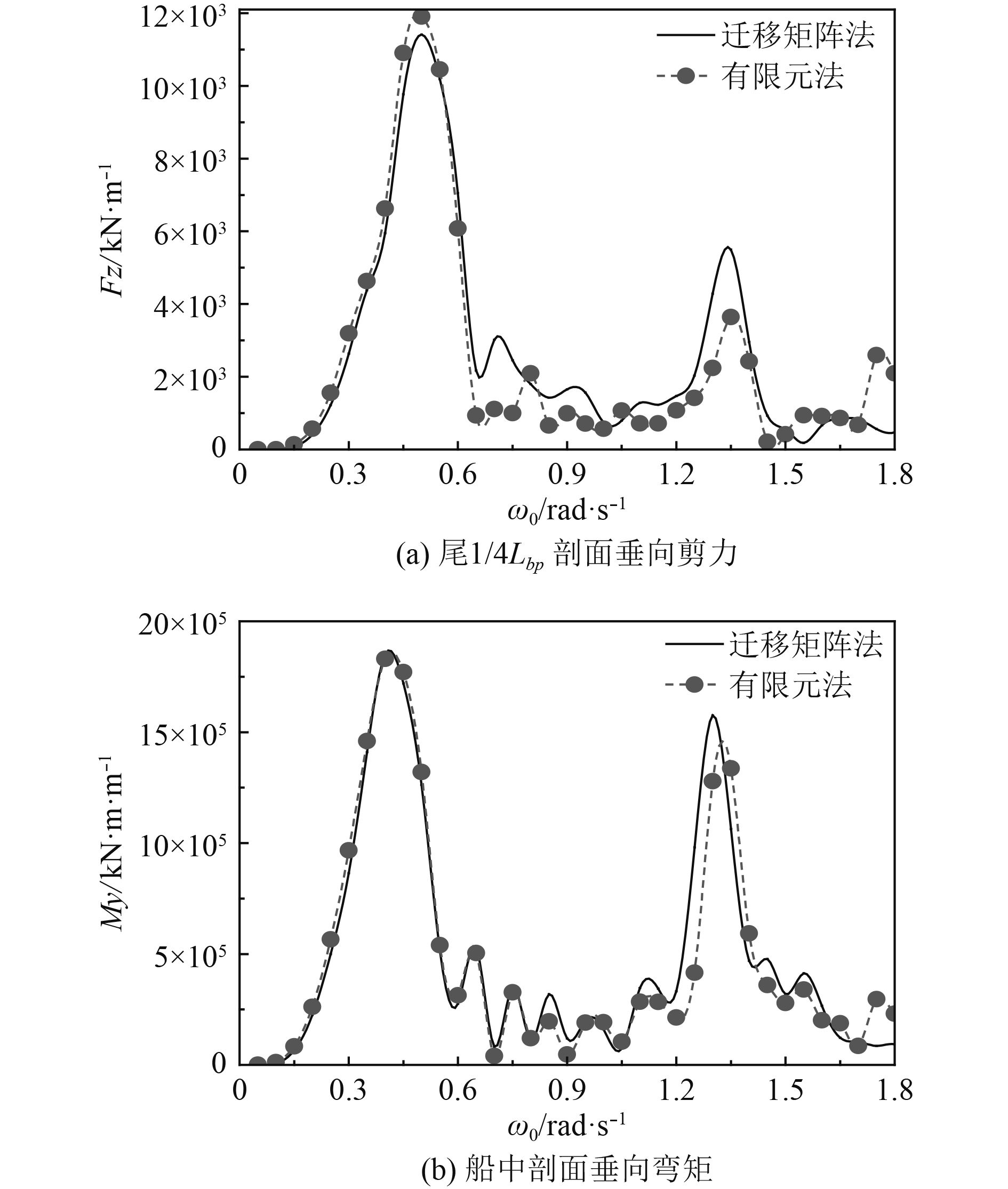图 7 垂向载荷数值结果对比分析 Fig. 7 Numerical comparison and analysis of vertical wave loads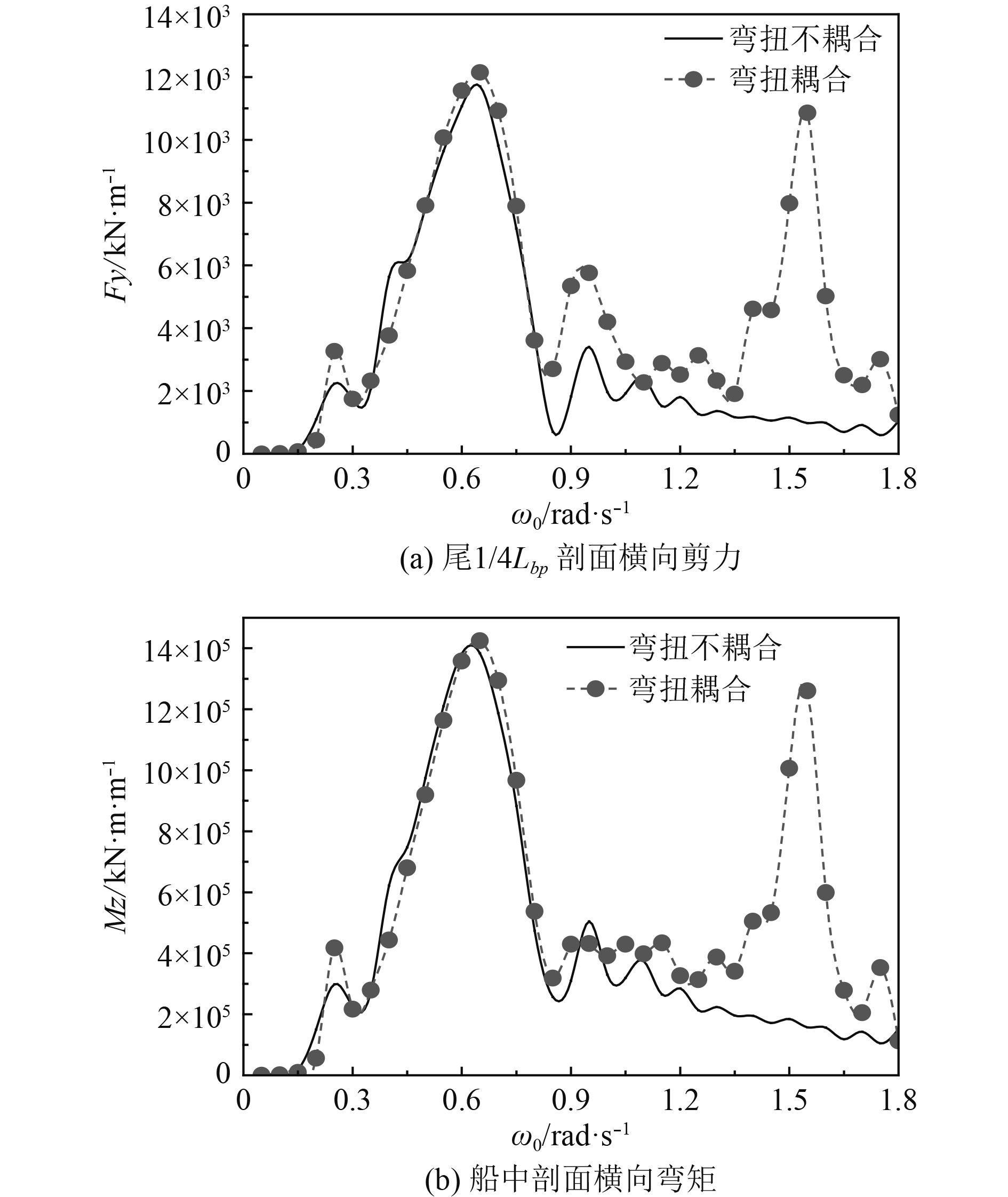图 8 水平载荷计算结果对比分析 Fig. 8 Numerical comparison and analysis of horizontal wave loads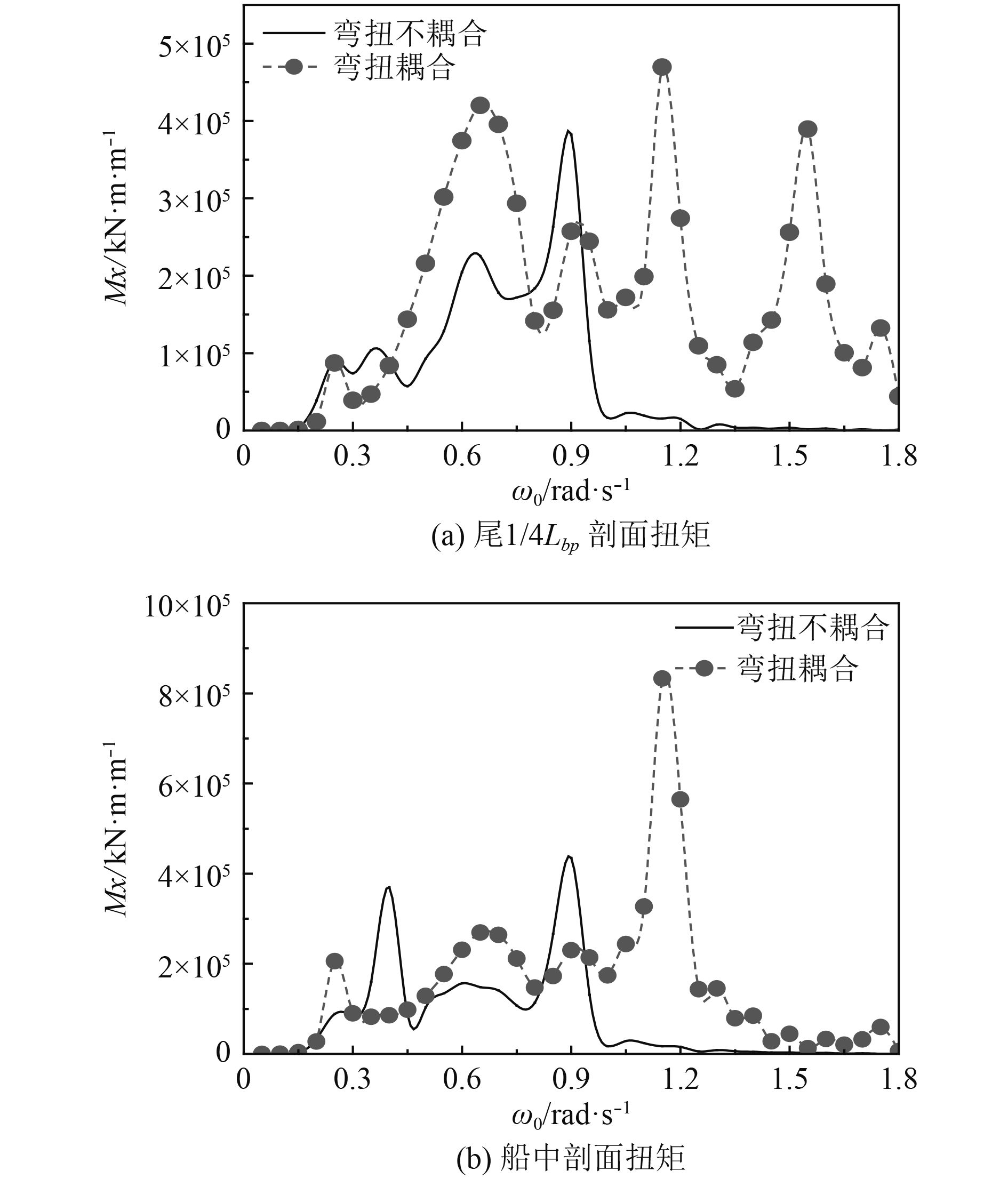图 9 扭矩数值结果对比分析 Fig. 9 Numerical comparison and analysis of torque
5 结　语

1）超大型集装箱船的抗扭转刚度很弱，不考虑弯扭耦合时计算得到的船体扭转固有频率相当低；

2）超大型集装箱船的弹性体效应十分明显，计算所得的波浪载荷的高频成分很明显，除扭矩的高频响应远大于低频响应外，其他载荷的高频弹振响应在数值上和低频响应处在同一水平；

3）对于超大型集装箱船来说，弯扭耦合模态对垂向载荷的数值计算结果无影响；

4）不考虑弯扭耦合效应时，水平载荷的高频响应数值结果不合理，而计算所得的纯扭转固有频率很低，导致扭矩中的弹振成分对应的频率和低频波浪激励扭矩所对应的频率十分接近，使得扭矩的弹振成分和低频部分在自然频率很低时就发生了重叠。

  MAIB. Report on the investigation of the structural failure of MSC Napoli[R]. English Channel on 18 January 2007, Marine Accident Investigation Branch (MAIB), UK, Report no. 9/2008. London, UK.  NK. Investigation report on structural safety of large container ships[R]. ClassNK, The investigative panel on large container ship safety, Tokyo, Japan, 2014.  Ivo Senjanović, Šime Malenica, Stipe Tomašević. Hydroelasticity of large container ships[J]. Marine Structures, 2008, 22(2): 287-314.  李辉. 船舶波浪载荷的三维水弹性分析方法研究[D]. 哈尔滨: 哈尔滨工程大学, 2009.  王迪. 弹振与颤振响应对超大型集装箱船的疲劳强度影响研究[D]. 哈尔滨: 哈尔滨工程大学. 2017.  MALENIA Š, MOLIN B, REMY F, SENJANOVIĆ I. Hydroelastic response of a barge to impulsive and non-impulsive wave load[C]// Proceedings 4th International Conference on Hydroelasticity in Marine Technology, Oxford, UK, pp. 107-15, 2003.  MALENIA Š, SENJANOVIĆ I, TOMAŠEVIĆ S. An efficient hydroelastic model for wave induced coupled torsional and horizontal ship vibrations[C]// In: 21st IWWWFB, UK, 2006. 113-16.  MALENIA Š, SENJANOVIĆ I, TOMAŠEVIĆ S, Stumpf E. Some aspects of hydroelastic issues in the design of ultra large container ships[C]// Proceedings 22nd International Workshop on Water Waves and Floating Bodies, Plitvice Lakes, Croatia, 2007.